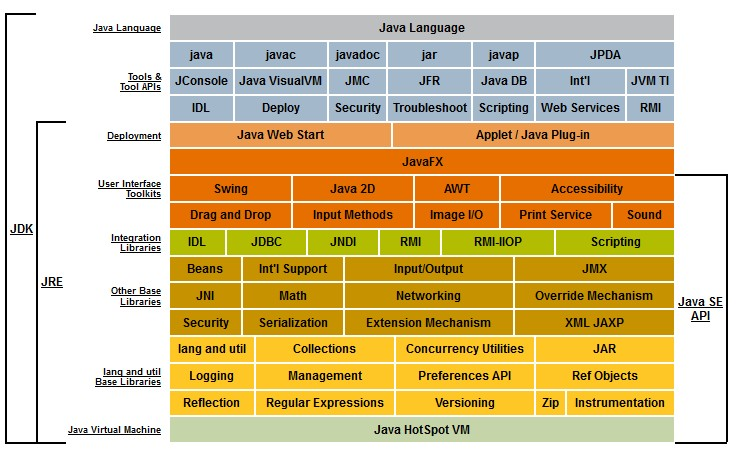# Computer Number - Float64 (64-bit or double precision) floating-point number

Float64 is a floating point number with a 64bit precision.

Float64 is also known as:

• 64-bit floating-point values,
• double precision floating-point
• 64-bit IEEE-754 floating-point
• or just double

It is a number bit representation that permits to store/manipulate:

in a computer

It's a logical data type. While your variable may have a float64 data type, the language engine will not store numbers in Float64 all the time because it will be terribly inefficient on a storage level.

## Language

• C: double. (implemented as a 64-bit IEEE 754 number, which uses 52 bits of space for the mantissa)
• Java: double

## Characteristic

### Storage

Storing numbers in Float64 would be terribly inefficient

Example: The integer number 42 in bit representation

representation bit
Float 64 0100 0000 0100 0101 0000 0000 0000 0000 0000 0000 0000 0000 0000 0000 0000 0000
Others
two’s complement 8-bit 0010 1010
two’s complement 32-bit 0000 0000 0000 0000 0000 0000 0010 1010
packed binary-coded decimal (BCD) 0100 0010
32-bit IEEE-754 floating-point 0100 0010 0010 1000 0000 0000 0000 0000

### Max / Min

• 53 bit - the max/min
``````console.log(Number.MAX_SAFE_INTEGER); // 2^53 - 1
console.log(Number.MIN_SAFE_INTEGER); // (-(2^53 - 1)).```
```

## Documentation / Reference

Discover MoreComputer - Double Number

DOUBLE is short name for the double-precision 64-bit floating point number.Computer - Floating-point (Representation and usage)

Computer representations of floating point numbers typically use a form of rounding to significant figures, but with binary numbers. The number of correct significant figures is closely related to the...Computer Storage - Integer Data Type (Binary Data)

In computer, integer are stored in word from 8 to 64 bit. Because CPU manipulates integer data type, they are also sometime called binary data type. Bit Length Two's complement signed Unsigned Float...Java - Double

Double in Java They implements the 64-bit precision IEEE 754 floating point Documentation: java/lang/Double Double is a subtype of number. Literal Double.parseDouble always uses a dot...Java - Number

in Java. The abstract class Number is the superclass of platform classes representing numeric values that are convertible to the primitive types byte, double, float, int, long, and short. The...
Javascript - Byte Array (ArrayBuffer / DataView)

To manipulate a byte array, in javascript, you have: a ArrayBuffer that represents an array: of fixed amount of bytes (one element = 1 byte) of binary element known as typed object : Int8 / Uint8:...
Javascript - Number (Double)

Most programming languages have several types of numeric data, but JavaScript gets away with just one. All numbers are and are stored as doubles. See also': 32 bit 53 bit - the max of double-precision...SQL - Data Type

The Data Type of a column in SQL. They are defined in the ANSI/ISO SQL standard but may vary from sql engine (database) to another. The below table shows you the history of the data type in the ANSI...